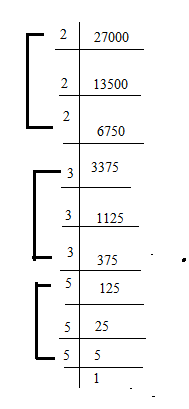QUESTION

# Find the cube root of 27000 by prime factorisation method.

HINT- Proceed the solution of this question by doing prime factor of given number then try to form triplets of prime numbers, if we get triplets of all prime numbers then it will be a perfect cube and multiplication of all prime numbers will be our cube root.

We know that,
The process of cubing is similar to squaring, only that the number is multiplied three times instead of two. The exponent used for cubes is 3. for examples are 8³ = 8*8*8 = 512.

In prime factorisation method-
we will find prime factors of 27000, if it is a perfect cube and then will pair them in a group of three.⇒27000=2×2×2×3×3×3×5×5×5
On forming pairs of three

⇒ 27000=${2^3} \times {3^3} \times {5^3}$

Since 2, 3 and 5 occurs in triplets
$\therefore$ 27000 is a perfect cube
⇒27000=${2^3} \times {3^3} \times {5^3}$

It can be write like,
⇒27000=${\left( {2 \times 3 \times 5} \right)^3}$

On taking cube root on both side
$\Rightarrow \sqrt{{\left( {27000} \right)}} = \sqrt{{{{\left( {2 \times 3 \times 5} \right)}^3}}}$

Cube root can be write raised to power $\frac{1}{3}$
$\Rightarrow \sqrt{{27000}} = {\left( {2 \times 3 \times 5} \right)^{3 \times \frac{1}{3}}}$

On cancelling exponential power
⇒ So cube root of 27000 ​= 2×3×5=30

Note- We should know that the cubic function is a one-to-one function. This is because cubing a negative number results in an answer different to that of cubing its positive counterpart. We can say this because when three negative numbers are multiplied together, two of the negatives are cancelled but one remains, so the result is also negative. 8³ = 8*8*8 = 512 and (-8) ³ = (-8) *(-8) *(-8) = -512.